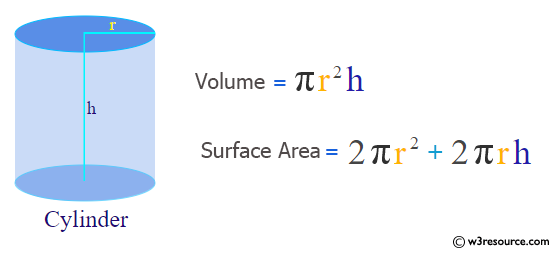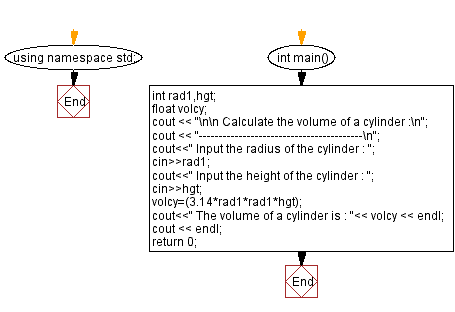﻿ C++ Exercises: Calculate the volume of a cylinder - w3resource

# C++ Exercises: Calculate the volume of a cylinder

## C++ Basic: Exercise-46 with Solution

Write a C++ program to calculate the volume of a cylinder.

Pictorial Presentation:Sample Solution:

C++ Code :

``````#include <iostream>
using namespace std;

int main()
{
float volcy;
cout << "\n\n Calculate the volume of a cylinder :\n";
cout << "-----------------------------------------\n";
cout<<" Input the radius of the cylinder : ";
cout<<" Input the height of the cylinder : ";
cin>>hgt;
cout<<" The volume of a cylinder is : "<< volcy << endl;
cout << endl;
return 0;
}
``````

Sample Output:

``` Calculate the volume of a cylinder :
-----------------------------------------
Input the radius of the cylinder : 4
Input the height of the cylinder : 8
The volume of a cylinder is : 401.92
```

Flowchart:C++ Code Editor:

What is the difficulty level of this exercise?

﻿

## C++ Programming: Tips of the Day

Why is there no std::stou?

The most pat answer would be that the C library has no corresponding "strtou", and the C++11 string functions are all just thinly veiled wrappers around the C library functions: The std::sto* functions mirror strto*, and the std::to_string functions use sprintf.

Ref: https://bit.ly/3wtz2qA

We are closing our Disqus commenting system for some maintenanace issues. You may write to us at reach[at]yahoo[dot]com or visit us at Facebook